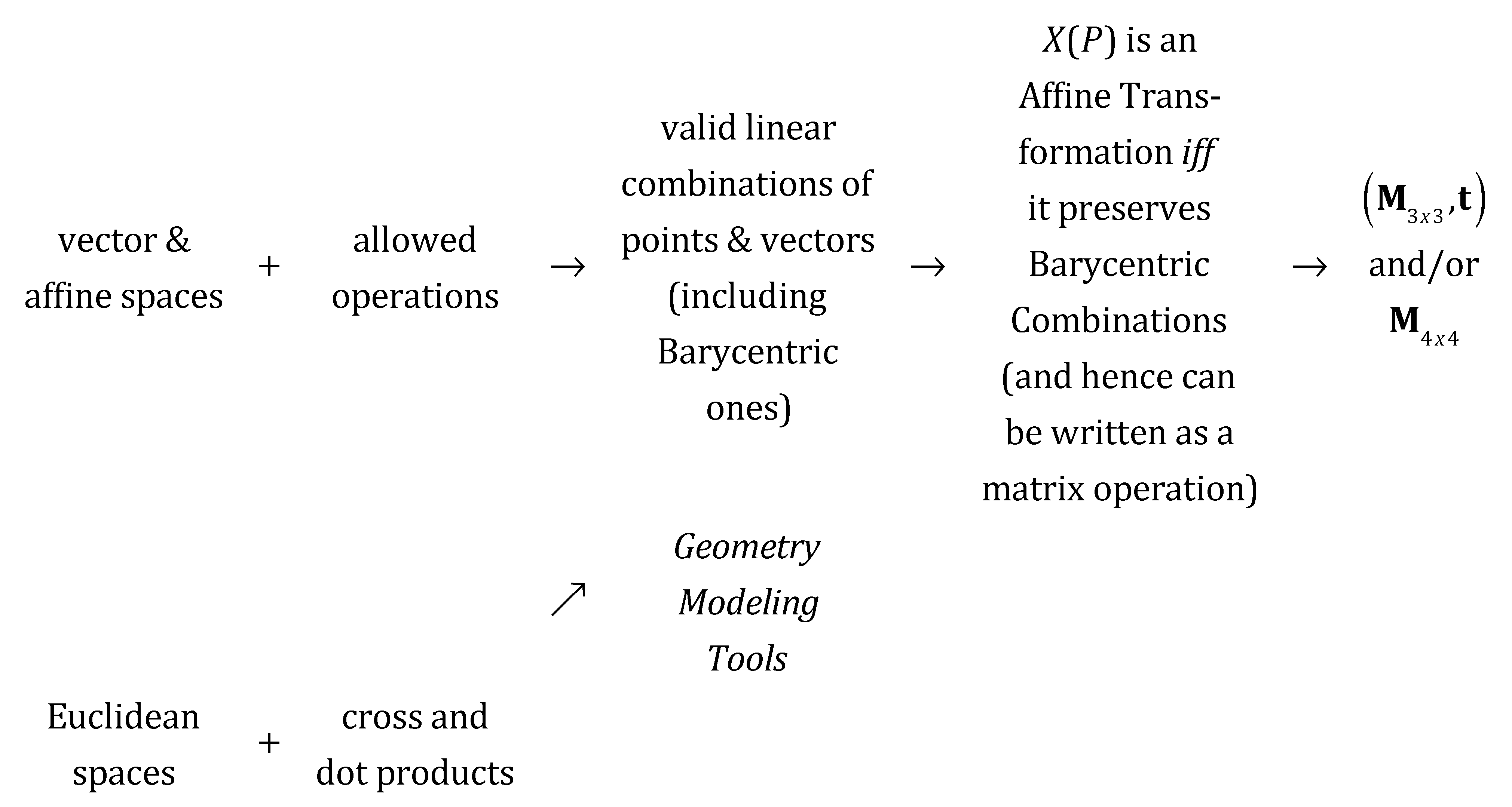# Affine Transformations: Basic Definitions and Concepts

### General linear combinations of points in an Affine Space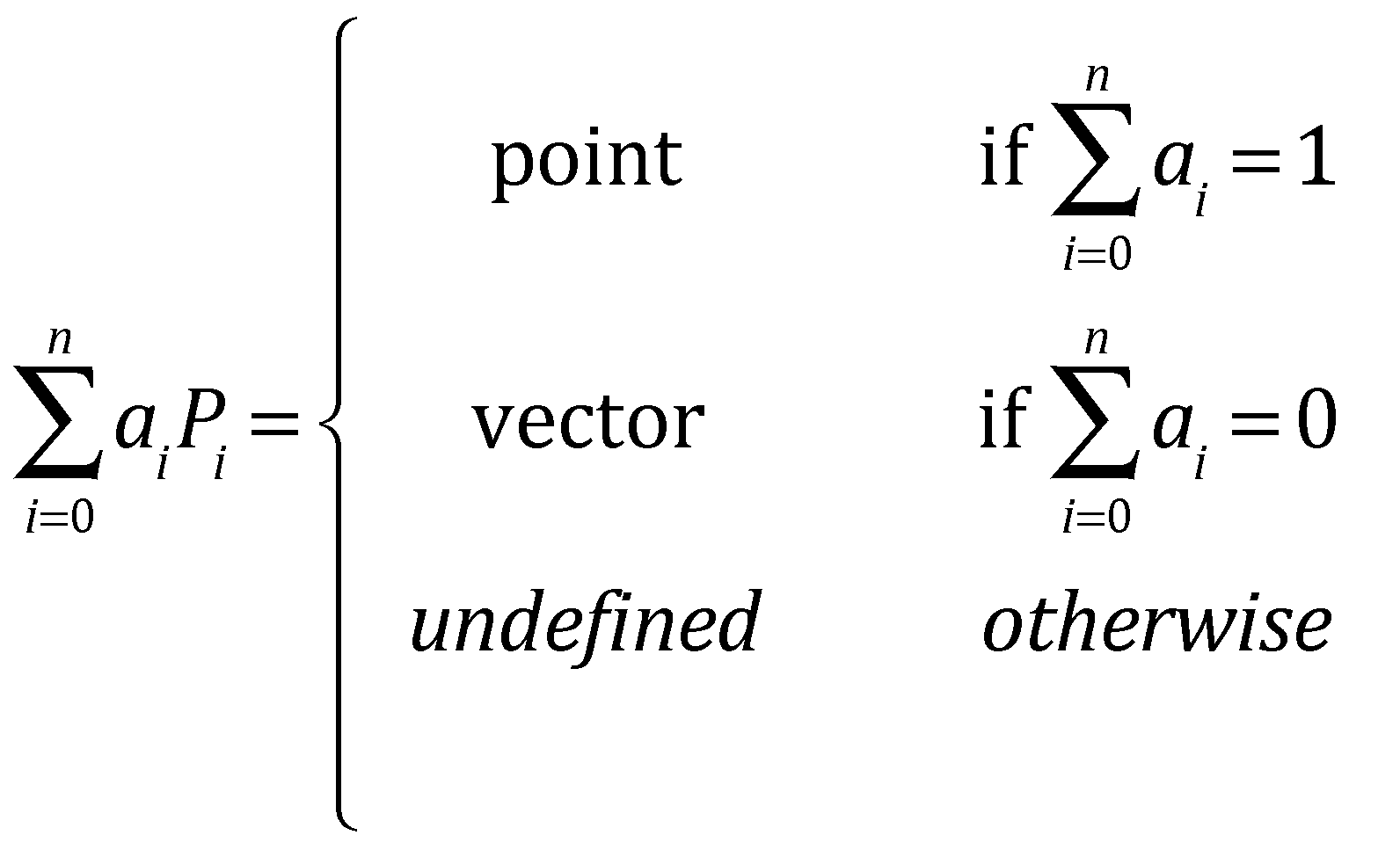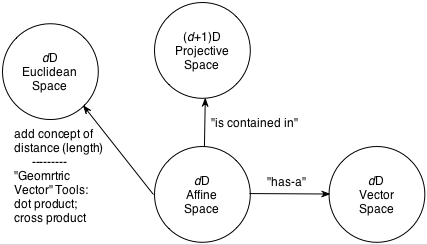1. Definition: A Barycentric Combination (or Barycentric Sum) is the special case of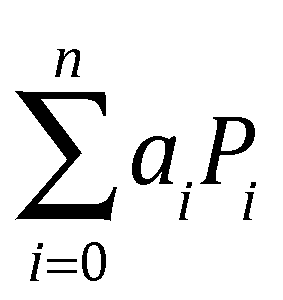in which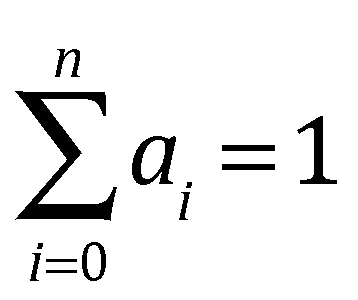.

In addition, if R is defined as the Barycentric combination: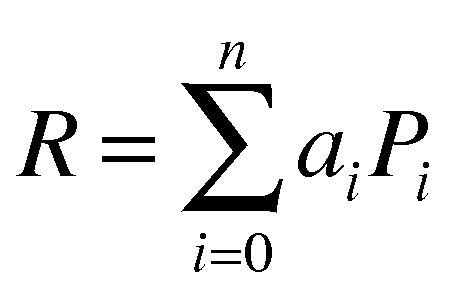then the ai are called the Barycentric coordinates of R with respect to the points Pi.

2. Definition: A Convex Combination (or Convex Sum) is a special case of Barycentric Combinations in which all ai ≥ 0.
3. Definition: An Affine Transformation is a mapping, X, from a point, Q in a d-dimensional affine space to another point Q′ in the same affine space that preserves Barycentric Combinations.

We will write this functionally as:

Q′ = X(Q)

### What Does "preserve Barycentric Combinations" Mean?

Given R defined as:X preserves Barycentric Combinations (and hence is an affine transformation) if and only if: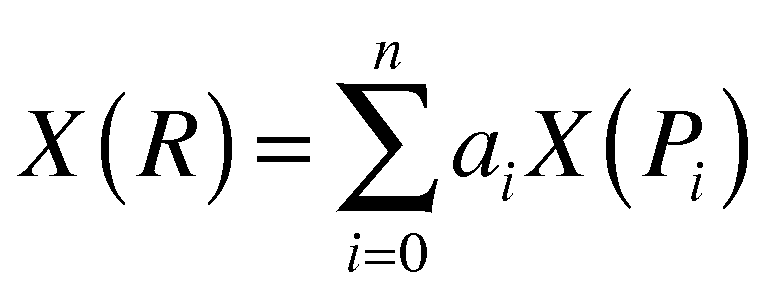1. With the exception of perspective projections, all transformations we will use in this course (e.g., mapping from Model Coordinates to Eye Coordinates as well as individual or concatenated transformations such as rotations, scales, mirrors, shears, and translations that may be applied to all or part of a model) are affine transformations.
2. So why are we interested in matrices?

It can be proven that X preserves Barycentric Combinations (and hence is an affine transformation on points in a d-dimensional affine space) if and only if it can be written as:

X(Q) = MQ + t
where:
• M is a d x d matrix that encodes all of the aspects of the transformation such as those listed above except the translation, and
• t is a d-dimensional vector that rigidly translates the oriented and scaled geometry

3. Why 4x4 matrices?

Creating and rendering 3D models is just a specific case of all this with d=3. In the equation for X(Q) in the previous item, M is a d x d matrix. Our interest is in 3D geometry (i.e., d=3), so let's be explicit and replace M with M3x3. Then we will embed our generic 3D point, Q, on the w=1 plane of projective space so that we can write: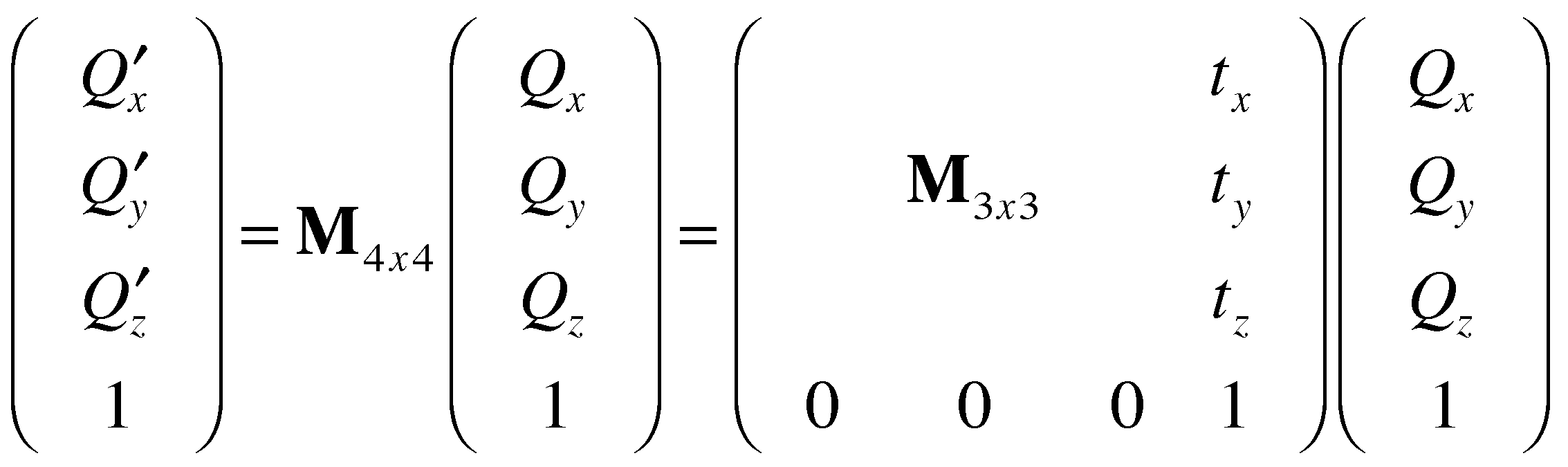Then X(Q) is just the first three elements of the left-hand side. Moreover, perspective projections (our "exception" from #1) can be represented using a 4x4 matrix with a bottom row different from (0, 0, 0, 1), so we have a representational form that can encode both affine and projective transformations.

4. Can affine transformations be applied to vectors? If so, how?
• Notice that the entire definition of affine transformations is based on points, and there is no mention of vectors.
• Affine transformations cannot be applied to vectors the same way as they are to points for (at least) two reasons:
• Vectors have no position, hence adding t would be meaningless and invalid.
• The way such a transformation should be applied to a vector depends on the relationship captured in the vector!
• Offset between two points: X(v) = v′ = Mv
• Orientation of a plane: X(v) = v′ = (M-1)Tv

## The Grand Summary